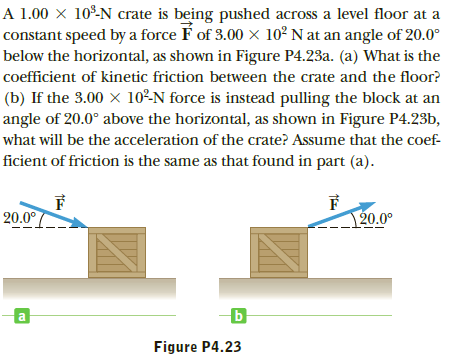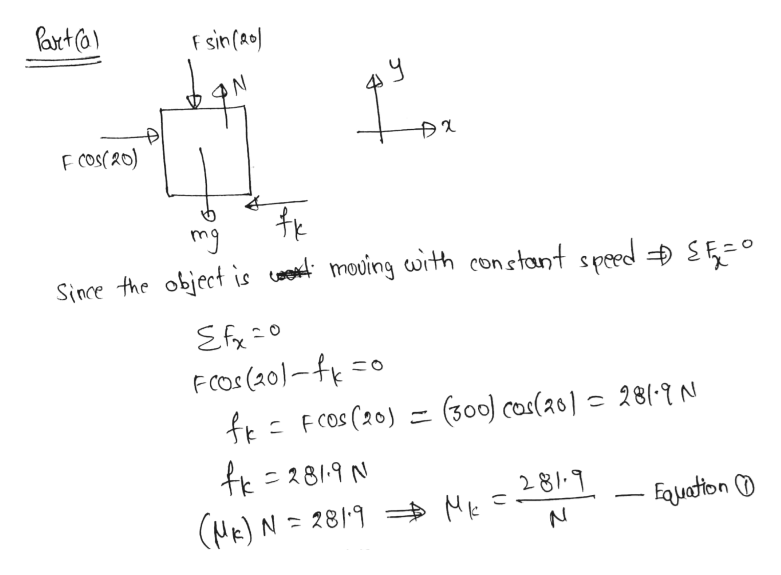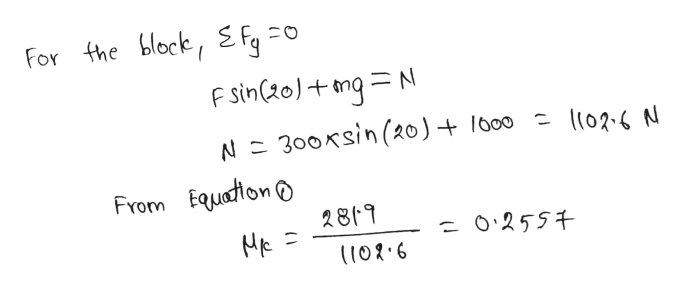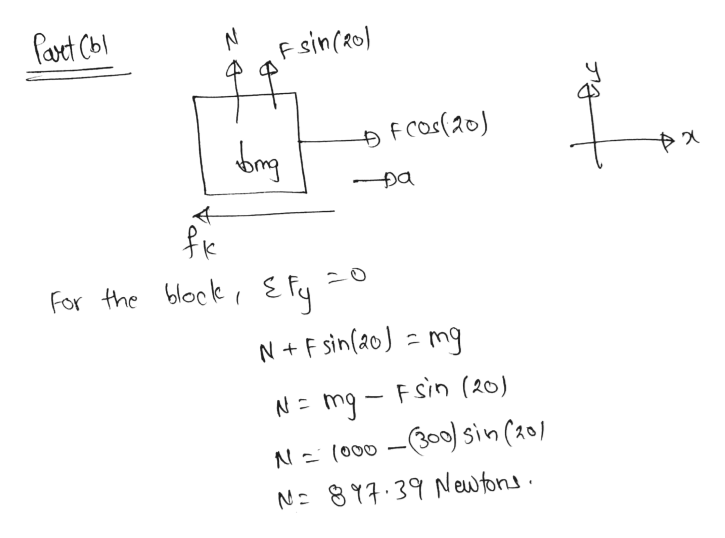# A 1.00 × 10º-N crate is being pushed across a level floor at aconstant speed by a force É of 3.00 x 10² N at an angle of 20.0°below the horizontal, as shown in Figure P4.23a. (a) What is thecoefficient of kinetic friction between the crate and the floor?(b) If the 3.00 × 10²N force is instead pulling the block at anangle of 20.0° above the horizontal, as shown in Figure P4.23b,what will be the acceleration of the crate? Assume that the coef-ficient of friction is the same as that found in part (a).20.0°120.0°Figure P4.23

Question
1 viewshelp_outlineImage TranscriptioncloseA 1.00 × 10º-N crate is being pushed across a level floor at a constant speed by a force É of 3.00 x 10² N at an angle of 20.0° below the horizontal, as shown in Figure P4.23a. (a) What is the coefficient of kinetic friction between the crate and the floor? (b) If the 3.00 × 10²N force is instead pulling the block at an angle of 20.0° above the horizontal, as shown in Figure P4.23b, what will be the acceleration of the crate? Assume that the coef- ficient of friction is the same as that found in part (a). 20.0° 120.0° Figure P4.23 fullscreen
check_circle

Step 1help_outlineImage TranscriptionclosePart (0) F sin (20) F cos(20) mg Since the object is corest moving with constant speed Đ SE=O Efx=0 Fcos (20)–fk = ft= Fcos (20) = (300) cor(20) c 28(-9 N fk=2819 N 281.9 Fojuation O (HE) N = 2819 \$ Mk fullscreen
Step 2help_outlineImage TranscriptioncloseFor the block, EFfy F sin(20)+mg=N N= 300xsin (20) + 100 = 1l02.6 N From Equation o 2819 = 02557 Mk = (102.6 fullscreen
Step 3help_outlineImage TranscriptioncloseParet Cbl F sin(20) F Cos(20) bing fk For the block, E fy N+ F sin(20) = mg N= mg - Fsin (20) N= (000 -(300) sin (201 N= 897.39N ew tons. fullscreen

### Want to see the full answer?

See Solution

#### Want to see this answer and more?

Solutions are written by subject experts who are available 24/7. Questions are typically answered within 1 hour.*

See Solution
*Response times may vary by subject and question.
Tagged in

### Physics